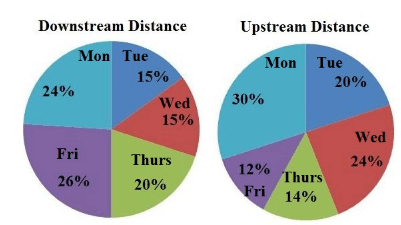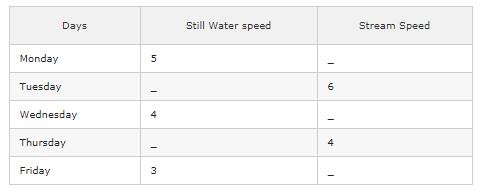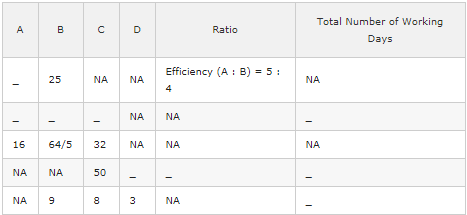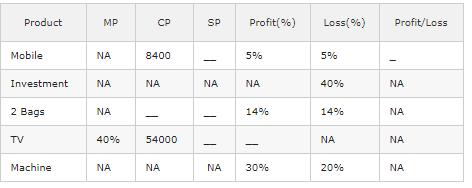Data Interpretation MCQ -3

# Data Interpretation MCQ -3

Test Description

## 20 Questions MCQ Test | Data Interpretation MCQ -3

Data Interpretation MCQ -3 for Banking Exams 2022 is part of Banking Exams preparation. The Data Interpretation MCQ -3 questions and answers have been prepared according to the Banking Exams exam syllabus.The Data Interpretation MCQ -3 MCQs are made for Banking Exams 2022 Exam. Find important definitions, questions, notes, meanings, examples, exercises, MCQs and online tests for Data Interpretation MCQ -3 below.
Solutions of Data Interpretation MCQ -3 questions in English are available as part of our course for Banking Exams & Data Interpretation MCQ -3 solutions in Hindi for Banking Exams course. Download more important topics, notes, lectures and mock test series for Banking Exams Exam by signing up for free. Attempt Data Interpretation MCQ -3 | 20 questions in 15 minutes | Mock test for Banking Exams preparation | Free important questions MCQ to study for Banking Exams Exam | Download free PDF with solutions
 1 Crore+ students have signed up on EduRev. Have you?
Data Interpretation MCQ -3 - Question 1

### Study the following graph carefully to answer the given questions Down Stream Distance = 200 km                      Up Stream Distance = 300 kmA boatman rows down stream at 6kmph on Wednesday. Find the speed of upstream and Speed of current?

Detailed Solution for Data Interpretation MCQ -3 - Question 1

Downstream Speed = 6kmph
Upstream Speed = 8kmph – 6kmph = 2kmph
Speed of Current = (6-2)/2 = 2kmph

Data Interpretation MCQ -3 - Question 2

### Study the following graph carefully to answer the given questions Down Stream Distance = 200 km                      Up Stream Distance = 300 kmA boatman rows up stream at 4kmph, 2 kmph and 8 kmph on Monday, Wednesday and Thursday respectively. Then find the ratio between the down stream speed of boat on Monday, Wednesday, and Thursday together and the rate of current on the same days together?

Detailed Solution for Data Interpretation MCQ -3 - Question 2

Downstream Speed of boat on Monday = 10 – 4 = 6kmph. similarly Downstream Speed of boat on Wednesday and Thursday = 6 kmph, 16kmph
Rate of current = 1kmph, 2kmph and 4kmph
Ratio = 28:7 = 4 : 1

Data Interpretation MCQ -3 - Question 3

### Study the following graph carefully to answer the given questions Down Stream Distance = 200 km                      Up Stream Distance = 300 kmA boatman rows up stream at 16 kmph and 2 kmph on Tuesday and Friday. Then find the difference between the down stream speed of boat on Tuesday and Friday together and the speed of boat in still water on the same days together?

Detailed Solution for Data Interpretation MCQ -3 - Question 3

down stream speed of boat on Tuesday and Friday = 32 kmph
Still water speed = 25 kmph
Difference = 32 – 25 = 7 kmph

Data Interpretation MCQ -3 - Question 4

Study the following graph carefully to answer the given questions

Down Stream Distance = 200 km                      Up Stream Distance = 300 kmWhat time will be taken by a boat to cover a distance on Tuesday along the stream, if the up stream of boat is 3 kmph?

Detailed Solution for Data Interpretation MCQ -3 - Question 4

upstream of boat = 3 kmph
down stream of boat =15 kmph
Down Stream Distance = 15% of 200 = 30; Time = 30/15 = 2 hr

Data Interpretation MCQ -3 - Question 5

Study the following graph carefully to answer the given questions

Down Stream Distance = 200 km                      Up Stream Distance = 300 kmWhat is the sum of total distance travelled by the boat on Monday, Wednesday and Friday together?

Detailed Solution for Data Interpretation MCQ -3 - Question 5

Total distance travelled by the boat on Monday = 48 + 90 = 138
Similarly Total distance travelled by the boat on Wednesday and Friday = 102, 88
Total distance travelled by the boat on Monday, Wednesday and Friday = 138 + 102 + 88 = 328 km

Data Interpretation MCQ -3 - Question 6

Study the following graph carefully to answer the given questions

Down Stream Distance = 200 km                      Up Stream Distance = 300 kmFind the ratio between the down stream distance travelled by boat on Monday, Wednesday, Thursday and Friday together and the Up stream distance travelled by the boat on the same days together?

Detailed Solution for Data Interpretation MCQ -3 - Question 6

Down stream distance travelled by boat on Monday, Wednesday, Thursday and Friday together = 48 + 30 + 40 + 52 = 170
Up stream distance travelled by boat on Monday, Wednesday, Thursday and Friday together = 90 + 72 + 42 + 36 = 240
17 : 24.

Data Interpretation MCQ -3 - Question 7

Study the following graph carefully to answer the given questions

Down Stream Distance = 200 km                      Up Stream Distance = 300 kmFind the difference between the up stream distance travelled by boat on Tuesday and Friday together and the down stream distance travelled by boat on the same days together?

Detailed Solution for Data Interpretation MCQ -3 - Question 7

Difference  = 96 – 82 = 14

Data Interpretation MCQ -3 - Question 8

Study the following graph carefully to answer the given questions

Down Stream Distance = 200 km                      Up Stream Distance = 300 kmTotal downstream distance travelled by boat on Monday, Tuesday and Wednesday together is what percentage of total upstream distance travelled by boat on the same days together?

Detailed Solution for Data Interpretation MCQ -3 - Question 8

Total downstream distance travelled by boat on Monday, Tuesday and Wednesday = 108
Total up stream distance travelled by boat on Monday, Tuesday and Wednesday = 222
% = 108/222 * 100 = 48.6%

Data Interpretation MCQ -3 - Question 9

Study the following graph carefully to answer the given questions

Down Stream Distance = 200 km                      Up Stream Distance = 300 kmA boatman rows down stream at 6kmph on Wednesday. Find the time taken by the boat to cover upsream distance on the same day?

Detailed Solution for Data Interpretation MCQ -3 - Question 9

Downstream Speed = 6kmph
Upstream Speed = 8kmph – 6kmph = 2kmph
Time taken by the boat to cover upstream distance = 72/2 = 36 hours

Data Interpretation MCQ -3 - Question 10

Study the following graph carefully to answer the given questions

Down Stream Distance = 200 km                      Up Stream Distance = 300 kmA boatman rows up stream at 16 kmph on Tuesday. Find the approximate time taken by the boat to cover downstream distance on the same day?

Detailed Solution for Data Interpretation MCQ -3 - Question 10

Downstream speed = 28 kmph; Time taken by the boat to cover downstream distance = 30/28 = 1 hr

Data Interpretation MCQ -3 - Question 11

(Q.no: 11-15). Refer to the table and answer the given questions.
NA refers the data which is not needed for a particular question. The number of working days of A, B, C, D is given below and some of them are missing. Take the data of the first row for the first question and the second row for the second question and so on.A started a work alone and then B joined her 5 days before actual completion of the work. For how many days A worked alone?

Detailed Solution for Data Interpretation MCQ -3 - Question 11

Efficiency (A : B) = 5 : 4
Number of days(A : B) = 4x : 5x = 4x : 25
∴ Number of days required by A to finish the work alone = 4x
= 4 x 5 = 20.
A and B work together for last 5 days = 5 x 9 = 45%
Efficiency of A = 5% and B’s efficiency = 4%
∴ No. of days taken by A to complete 55% work = 55/5 = 11days

Data Interpretation MCQ -3 - Question 12

(Q.no: 11-15). Refer to the table and answer the given questions.
NA refers the data which is not needed for a particular question. The number of working days of A, B, C, D is given below and some of them are missing. Take the data of the first row for the first question and the second row for the second question and so on.Working together B and C take 50% more number of days than A, B and C together take and A and B working together, take 8/3 more number of days than A, B and C take together. If A, B and C all have worked together till the completion of the work and B has received Rs.120 out of total earnings of Rs. 480 then in how many days did A, B and C together complete the whole work?

Detailed Solution for Data Interpretation MCQ -3 - Question 12

The days ratio of (A + B + C) : (B + C) = X:3X/2 = 2X:3x;
Efficiency ratio = 3X:2X
Efficiency of A = x.
(480/3X) = Rs.160
Amount received by B = Rs.120 & C = 200
160:120:200 =4:3:5
1/4:1/3:1/5= 15:20:12;
(1/15+1/12+1/20)*Y = 1
Y = 5 days

Data Interpretation MCQ -3 - Question 13

(Q.no: 11-15). Refer to the table and answer the given questions.
NA refers the data which is not needed for a particular question. The number of working days of A, B, C, D is given below and some of them are missing. Take the data of the first row for the first question and the second row for the second question and so on.All of them started to work together but A leaves after 4 days. B leaves the job 3 days before the completion of the work. How long would the work last?

Detailed Solution for Data Interpretation MCQ -3 - Question 13

Let the work lasted for x days,
A’s 4 day’s work + B (x – 3) day’s work + C’s x day’s work = 1
⇒ (4/16) + (x – 3) / (64/5) + x/32 = 1
⇒ 5(x – 3)/64 + x/32 = 1 – 1/4
⇒ [5(x – 3) + 2x] / 64 = 3/4
⇒ 7x – 15 = 48
∴ x = (48 + 15)/7 = 63/7 = 9 days

Data Interpretation MCQ -3 - Question 14

(Q.no: 11-15). Refer to the table and answer the given questions.
NA refers the data which is not needed for a particular question. The number of working days of A, B, C, D is given below and some of them are missing. Take the data of the first row for the first question and the second row for the second question and so on.C started the work and left after some days, when 25% work was done. After it D joined and completed it working for 25 days. In how many days C and D can do the complete work, working together?

Detailed Solution for Data Interpretation MCQ -3 - Question 14

Efficiency of C = (100/50) = 2%
Rest work = 75%
∴ Efficiency of D = 75/25 = 3%
∴ Combined efficiency of C and D = 5%
∴ Number of days required by C and D to work together = 100/5 = 20 days.

Data Interpretation MCQ -3 - Question 15

(Q.no: 11-15). Refer to the table and answer the given questions.
NA refers the data which is not needed for a particular question. The number of working days of A, B, C, D is given below and some of them are missing. Take the data of the first row for the first question and the second row for the second question and so on.B and C started the work. After 3 days D joined them. What is the total number of days in which they had completed the work?

Detailed Solution for Data Interpretation MCQ -3 - Question 15

Efficiency of B and C = 11.11 + 5.55 = 16.66%
Work done in 3 days = 3 x 16.66 = 50%
Rest work done by B, C and D = 50/50 = 1 day
Work can be completed in 4 days.

Data Interpretation MCQ -3 - Question 16

(Q.no: 16 -20). Refer to the table and answer the given questions.

NA refers the data which is not needed for a particular question. The price details of 5 products are given below and some of them are missing. Take the data of the first row for the first question and the second row for the second question and so on.Rahul purchased a mobile and sold it for a loss(loss % given in the table). From that money, he purchased another article and sold it for a gain of (Profit % given in the table). What is the overall gain or loss?

Detailed Solution for Data Interpretation MCQ -3 - Question 16

CP = 8400
SP = 8400 * 95/100 = 7980
CP = 7980
SP = 7980 * 105/100 = 8379
Difference = 8400 – 8379 = 21

Data Interpretation MCQ -3 - Question 17

(Q.no: 16 -20). Refer to the table and answer the given questions.

NA refers the data which is not needed for a particular question. The price details of 5 products are given below and some of them are missing. Take the data of the first row for the first question and the second row for the second question and so on.A, B and C invests rupees 8000, 12000 and 10000 respectively in a business. At the end of the year the balance sheet shows a loss of initial investment. Find the share of loss of B.

Detailed Solution for Data Interpretation MCQ -3 - Question 17

Total loss after one year = 30000*40/100 = 12000
share of B = (40/10)*12000 = 4800

Data Interpretation MCQ -3 - Question 18

(Q.no: 16 -20). Refer to the table and answer the given questions.

NA refers the data which is not needed for a particular question. The price details of 5 products are given below and some of them are missing. Take the data of the first row for the first question and the second row for the second question and so on.A Shop Keeper sells two bags for Rs. 500 each. On one, he gets % profit(as per the table) and on the other he gets % loss(as per the table). His profit or loss in the entire transaction was?

Detailed Solution for Data Interpretation MCQ -3 - Question 18

% = x
Loss % = x²/100 = 196/100 = 49/25%

Data Interpretation MCQ -3 - Question 19

(Q.no: 16 -20). Refer to the table and answer the given questions.

NA refers the data which is not needed for a particular question. The price details of 5 products are given below and some of them are missing. Take the data of the first row for the first question and the second row for the second question and so on.The price of TV was marked up by %(as per the table).It was sold at a discount of 20% on the marked price. What was the profit percent of the cost price?

Detailed Solution for Data Interpretation MCQ -3 - Question 19

40 – 20 + [40 * (-20)/100] = 20 – 8 = 12%

Data Interpretation MCQ -3 - Question 20

(Q.no: 16 -20). Refer to the table and answer the given questions.

NA refers the data which is not needed for a particular question. The price details of 5 products are given below and some of them are missing. Take the data of the first row for the first question and the second row for the second question and so on.Preethi sold a machine to Shalini at a profit of %(as per table). Shalini sold this machine to Arun at a loss of %(as per table). If Preethi paid Rs.5000 for this machine, then find the cost price of machine for Arun?

Detailed Solution for Data Interpretation MCQ -3 - Question 20

R1 = 30% R2 = 20%
5000 * 130/100 * 80/100 = Rs. 5200

 Use Code STAYHOME200 and get INR 200 additional OFF Use Coupon Code
Information about Data Interpretation MCQ -3 Page
In this test you can find the Exam questions for Data Interpretation MCQ -3 solved & explained in the simplest way possible. Besides giving Questions and answers for Data Interpretation MCQ -3, EduRev gives you an ample number of Online tests for practice